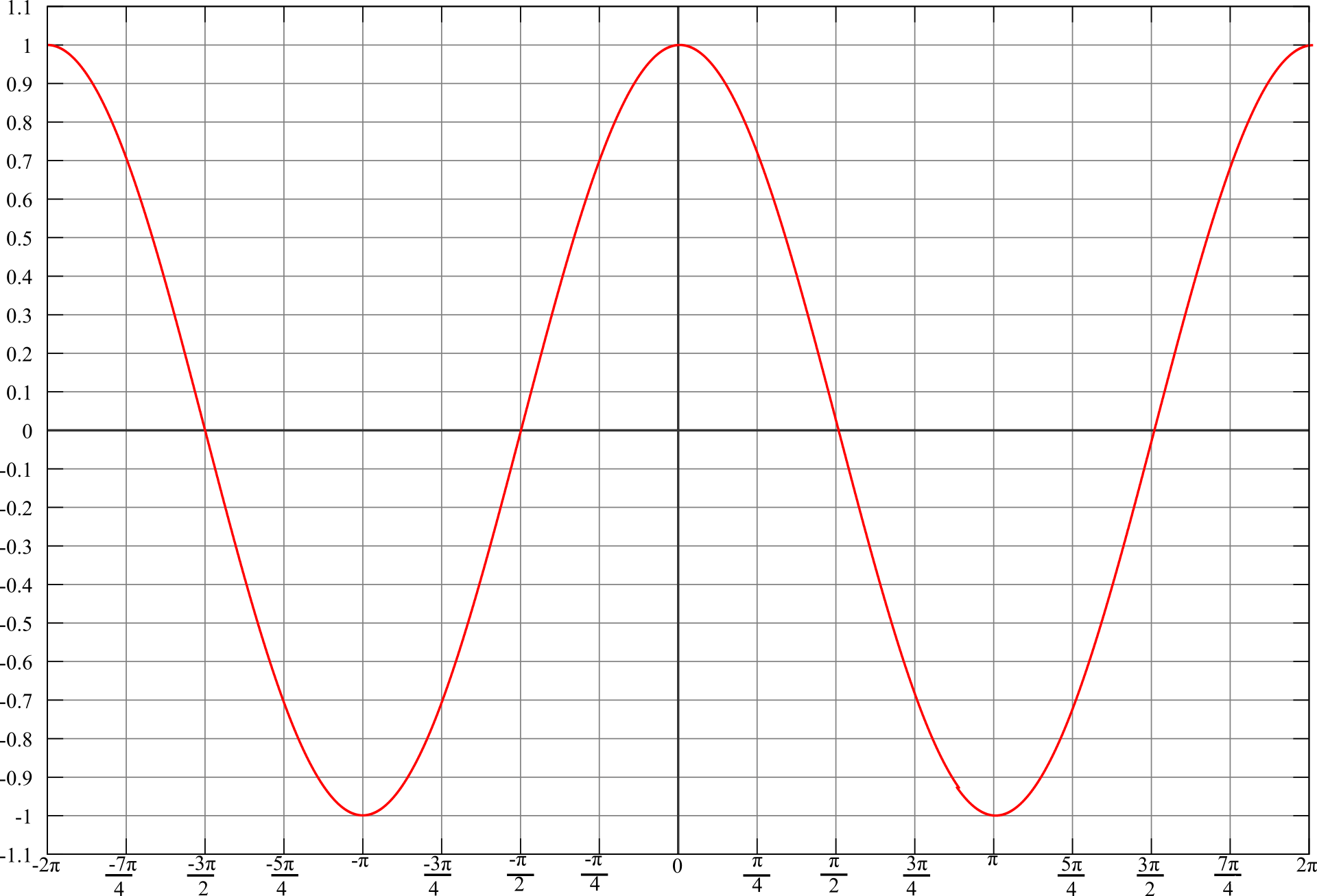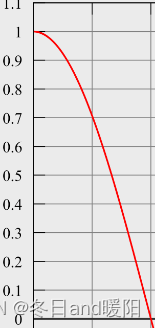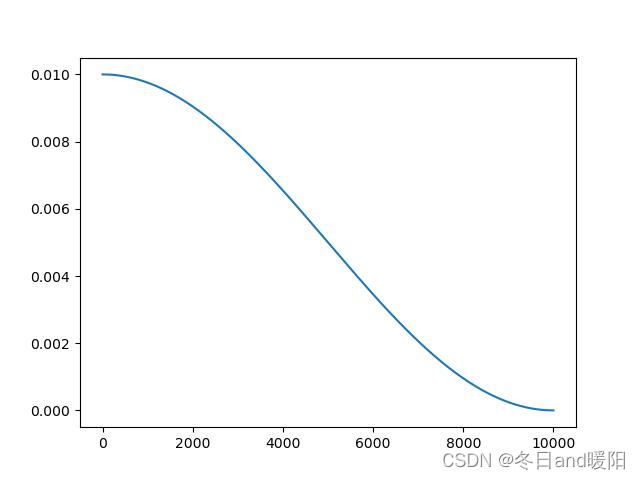# 二、余弦退火的目的和用法

## 2.1 为啥用cosineAnnealingLR策略``````torch.optim.lr_scheduler.CosineAnnealingLR(optimizer, T_max, eta_min=0, last_epoch=- 1, verbose=False
``````

``

## 2.2 如果 希望 learning rate 每个epoch更新一次

``````import numpy as np
import torch
from torchvision import models
import matplotlib.pyplot as plt

net = models.resnet18(pretrained=False)

max_epoch=50 # 一共50 epoch
iters=200    # 每个epoch 有 200 个 bach

optimizer = torch.optim.SGD(net.parameters(), lr=0.01, momentum=0.9)
scheduler =  torch.optim.lr_scheduler.CosineAnnealingLR(optimizer = optimizer,
T_max =  max_epoch) #  * iters

lr = []
for epoch in range(max_epoch):
for batch in range(iters):
optimizer.step()

lr.append(scheduler.get_lr())
scheduler.step() # 注意 每个epoch 结束， 更新learning rate

plt.plot(np.arange(len(lr)), lr)
plt.savefig('aa.jpg')

``````## 2.3 每个batch 迭代都改变学习率

``````max_epoch=50 # 一共50 epoch
iters=200    # 每个epoch 有 200 个 bach

optimizer = torch.optim.SGD(net.parameters(), lr=0.01, momentum=0.9)
scheduler =  torch.optim.lr_scheduler.CosineAnnealingLR(optimizer = optimizer,
T_max =  max_epoch * iters ) #  调整了四分之一周期的长度

lr = []
for epoch in range(max_epoch):
for batch in range(iters):
optimizer.step()

lr.append(scheduler.get_lr())
scheduler.step() # 注意 每个batch 结束， 更新learning rate
``````